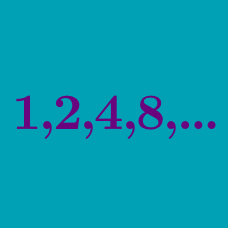Algebra

# Geometric Progressions

Given that the first few terms of a geometric progression are $$6, 18, 54, 162 \ldots$$, what is the common ratio of the geometric progression?

Given that the first, second, and third terms of a geometric progression are:

$3, 9, 27 ... ,$

what is the fifth term?

In the geometric sequence $\{ a_i \}_{i \geq 1} = \{ -7, 14, -28, \ldots, \}$ what is the value of $$n$$ such that $$a_n = 224?$$

If the six numbers

$7, k_1, k_2, k_3, k_4, \frac{7}{32}$

form a geometric sequence in this order, what is $$k_2?$$

Given that the third, fourth, and fifth terms of a geometric progression are:

$\ldots, 45, 135, 405, \ldots,$

what is the second term?

×